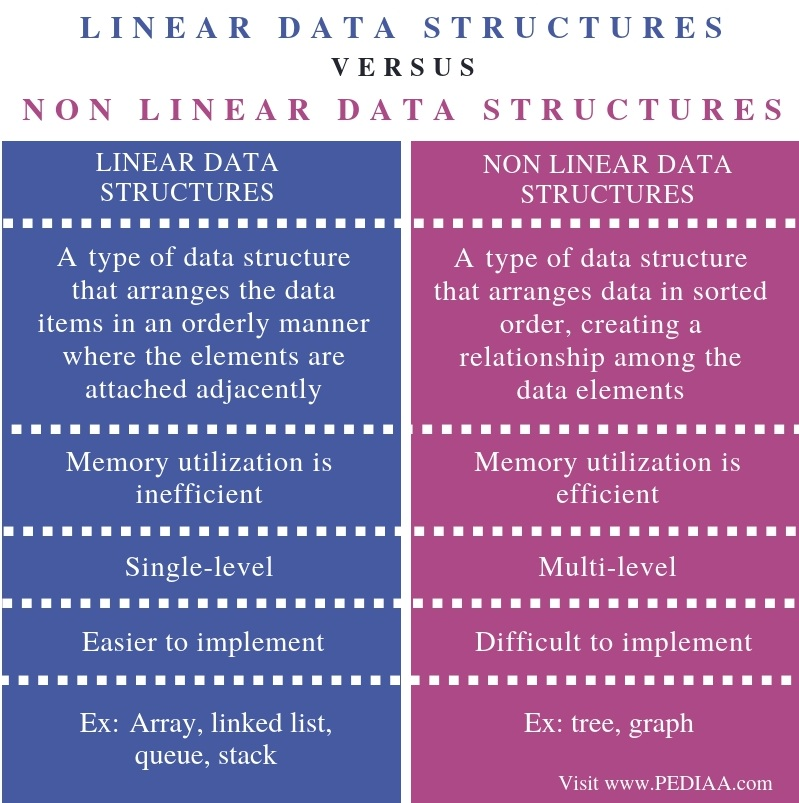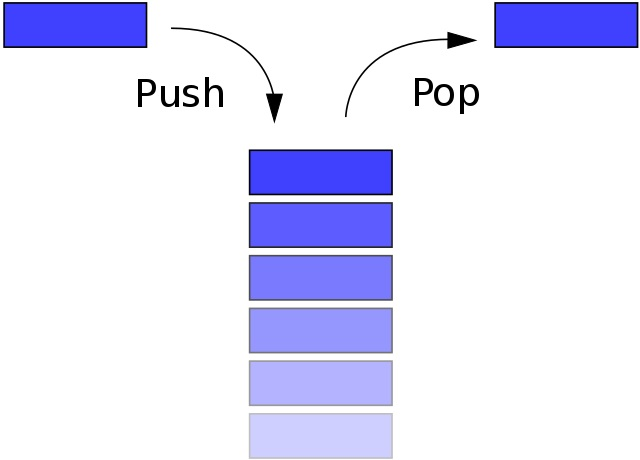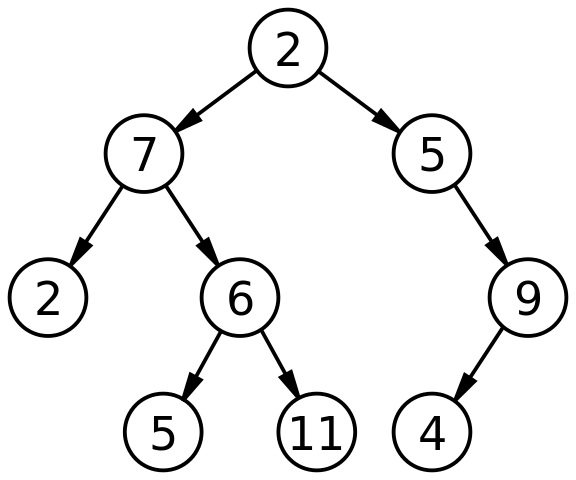What is the Difference Between Linear and Non Linear Data Structures

The main difference between linear and non linear data structures is that linear data structures arrange data in a sequential manner while nonlinear data structures arrange data in a hierarchical manner, creating a relationship among the data elements.

A data structure is a way of storing and managing data. There are two types of data structures as linear and nonlinear data structures. Linear data structures allow traversing through the items sequentially. On the other hand, in a nonlinear data structure, each element is attached to one or more elements creating a relationship among the items.

Key Areas Covered

1. What are Linear Data Structures
– Definition, Functionality, Examples
2. What are Non Linear Data Structures
– Definition, Functionality, Examples
3. What is the Difference Between Linear and Non Linear Data Structures
– Comparison of Key Differences

Key Terms

Linear Data Structures, Nonlinear Data StructuresWhat are Linear Data Structures

A linear data structure is a data structure that has data elements in sequential order. In a linear data structure, the adjacent elements are attached to each other. However, these data structures do not make better utilization of memory. Therefore, it can lead to memory wastage.

Array, Linked List, Stack, and Queue are some common examples of linear data structures. An array stores data elements of the same data type. A linked list is a data structure that contains a set of nodes; each node stores data and address of another node. These nodes connect together to form a structure similar to a chain.

A stack is an abstract data type that behaves similarly to a real-world stack. It follows LIFO (Last in First Out) order. In other words, the inserted last element is in the top of the stack. Therefore, the element to access the first is the last inserted element. Moreover, inserting elements to the stack is called push operation and removing elements from the stack is called pop operation.Figure 1: Linear data structure – Stack

A queue is also an abstract data structure, but it has two ends to insert elements and to delete elements. Inserting elements is called enqueue and removing elements is called dequeue.

What are Non Linear Data Structures

Non Linear data structure stores data in a non-sequential manner. It forms a hierarchical relationship among the child elements and parent elements. In other words, the data items are attached to each other creating a relationship between them. It is not possible to insert elements, delete elements or go through the elements in sequential order. Usually, these data structures are more memory efficient.

Trees and graphs are the most common nonlinear data structures. A tree data structure represents the nodes connected by edges. A binary tree is a tree data structure.  Every node in the binary tree can have a maximum of two children.Figure 2: Nonlinear Data Structure – Binary Tree

For example, in the above binary tree, 2 is the parent node while 7 and 5 are the child nodes. Furthermore, a graph is another data structure which consists of nodes and edges.

Difference Between Linear and Non Linear Data Structures

Definition

A linear data structure is a type of data structure that arranges the data items in an orderly manner where the elements are attached adjacently while a nonlinear data structure is a type of data structure that arranges data in sorted order, creating a relationship among the data elements. Thus, this is the main difference between linear and non linear data structures.

Memory Utilization

Memory utilization is inefficient in linear data structures while memory utilization is efficient in nonlinear data structures. Hence, this is another difference between linear and non linear data structures.

Levels

Furthermore, linear data structures are single-level while nonlinear data structures are multi-level.

Implementation

Implementation is one more difference between linear and non linear data structures. It is easier to implement linear data structures, but it is difficult to implement nonlinear data structures.

Examples

Array, linked list, queue, and stack are some examples of linear data structures while tree and graph are some examples of nonlinear data structures. This is another difference between linear and non linear data structures.

Conclusion

Data structures help to organize data in order to use them efficiently. There are mainly two types of data structures as linear and nonlinear data structures. The main difference between linear and non linear data structures is that linear data structures arrange data in a sequential manner while nonlinear data structures arrange data in a hierarchical manner creating a relationship among the data elements.

Reference:

1. “Tree – Javatpoint.” Www.javatpoint.com, Available here.
2. “DS Graph – Javatpoint.” Www.javatpoint.com, Available here.

Image Courtesy:

1. “Data stack” By User:Boivie – made in Inkscape, by myself User:Boivie. Based on Image:Stack-sv.png, originally uploaded to the Swedish Wikipedia in 2004 by sv:User:Shrimp (Public Domain) via Commons Wikimedia
2. “Binary tree” By Derrick Coetzee – Own work (Public Domain) via Commons Wikimedia